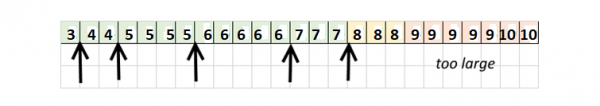# Height Limited Huffman Trees in Data Structure

Data Structure AlgorithmsAnalysis of AlgorithmsAlgorithms

The diagram of height limited or depth limited Huffman tree is given belowTree depth limitation is a non-trivial issue that most real-world Huffman implementations must deal with.

Huffman construction doesn't limit the height or depth. If it would, it is not possible for it to be "optimal". Granted, the largest depth of a Huffman tree is bounded by the Fibonacci series, but that leave sufficient room for larger depth than wanted.

What is the reason to limit Huffman tree depth? Fast Huffman decoders implement lookup tables. It's possible to implement multiple table levels to mitigate the memory cost, but a very fast decoder such as Huff0 goes for a single table, both for simplicity and speed. In which case the table size is treated as a direct product of the tree depth (tablesize = 1 <<treeDepth).

For the benefit of speed and memory management, a limit had to be chosen: it's 8 KB for the decoding table, which nicely fits into Intel's L1 cache, and leaves some room to combine it with other tables if need be. Since latest decoding table uses 2 bytes per cell, it translates into 4K cells, hence a maximum tree depth of 12 bits.

12 bits for compressing literals is generally too short, at least according to optimal Huffman construction.

Constructing a depth-limited tree is therefore a practical issue to solve.

Depth-limited Huffman trees have been studied since the 1960's, so there is so many literatures available.